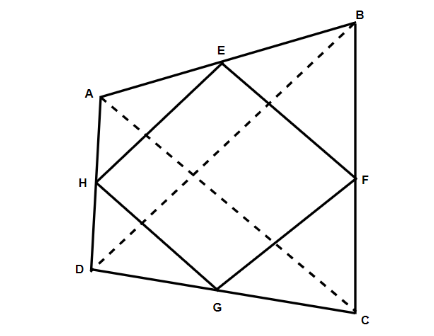QuestionAnswers

# $ABCD$ is a quadrilateral with unequal sides, unequal diagonals, and unequal angles. $E,F,G,H$ are the middle points of the four sides, then $EFGH$ is a: $\left( a \right){\text{ Quadrilateral with unequal sides}}$  $\left( b \right){\text{ Parallelogram}}$  $\left( c \right){\text{ Rhombus}}$  $\left( d \right){\text{ Rectangle}}$Verified
91.8k+ views
Hint: For solving this, we will first make the figure. And then taking the triangles made in then and comparing the lines we will get the lines are getting parallel to each other. And by using this we will conclude to answer this question.

Since it is given that the $ABCD$ is a quadrilateral with unequal sides and $E,F,G,H$ are the middle points of the four sides. So on joining the diagonals of the quadrilateral which will be named as $BD$ and $AC$ .Now we will take a triangle $ABD$ , for the reference we take it from the figure. So in this triangle $H$ and $E$ will be the midpoints of the sides $AD$ and $AB$ respectively.
Hence, from this we have $HE||BD$ .
Similarly, we will take a triangle $BCD$ , in this triangle $FG||BD$ and in the same way we will get $HE||GF$ .
Similarly, on considering the triangle $ABC$ and the triangle $ACD$ , we will get $EF||AC$ and $GH||CA$ . So from this, we can see that the quadrilateral $EFGH$ , in this the opposite sides are parallel. And we know that this is the property of parallelogram.
Therefore, the option $\left( b \right)$ is correct.